# Python Module

## Introduction

Modules are a simple way to organize a program which contains program code, variables etc.. All these definitions and statements are contained in a single Python file. The name of the module is the name of the file name with .py extension. Modules are not loaded unless we execute in Python interpreter or call within a program. In Python, there are modules in a standard library, current directory or directories containing .py files (in fact each file with .py extension is a module). To define a module, you can use Python IDLE, Notepad++ or any suitable text editor. Lets create a file called factorial.py which will create the factorial (in mathematics, the factorial of n [a positive integer] is the product of all positive integers less than or equal to n.) of a positive integer as well as some other jobs in the current directory.

Example:

``````# factorial.py
def factcal(n): # Create the factorial of a positive integer
fact = 1
while n>0:
fact *= n
n=n-1
if(n<=1):
break
else: # Display the message if n is not a positive integer.
print('Input a correct number....')
return
return fact

def factdata(n): # return the numbers of factorial x
result = []
while n>0:
result.append(n)
n = n - 1
if(n==0):
break
else: # Display the message if n is not a positive integer.
print('Input a correct number....')
return
return result
``````

Importing a module:

In order to use a module, use import statement. Go to the Python interpreter and execute the following command :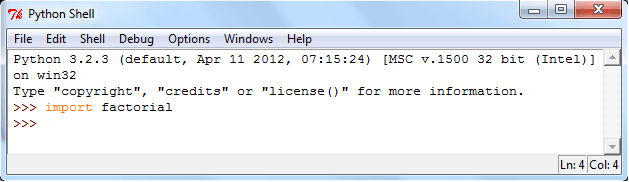There are two functions factcal(n) and factdata(n) are defined in factorial.py. Using the module name we can access the functions. functions factcal(5) creates the factorial of 5 and factdata(5) shows the numbers involved in factorial 5.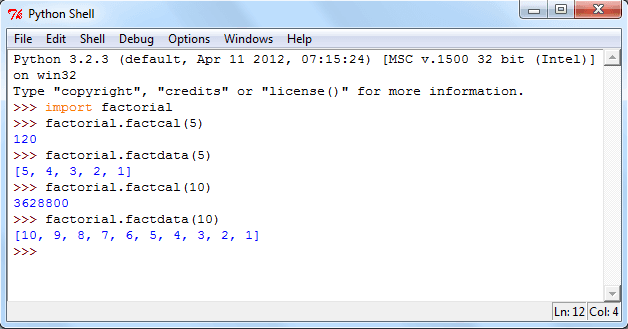In the above example instead of factorial.factcal(n) or factorial.factdata(n) we can assign it to a local name. See the following codes, we assign factorial.factcal into fumber and factorial.factdata into fdata.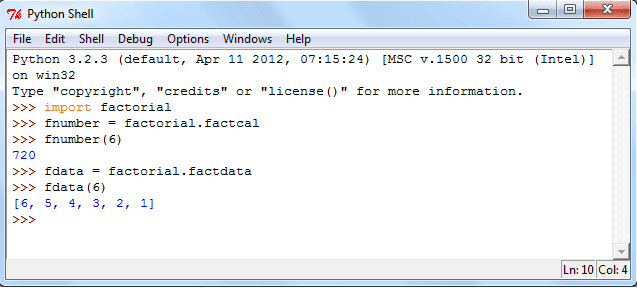In Python, a module name (as a string) is stored within a module which is available as the value of the global variable __name__.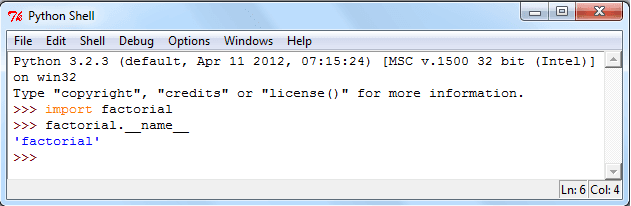from..import statement

from .. import statement is used to import selective names(s) or all names that a module defines. See the following codes.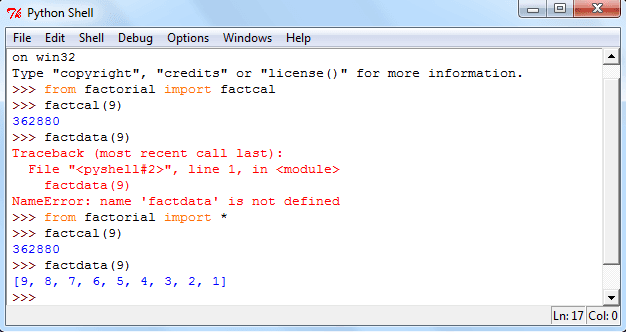In above example when we execute factdata(9), an error arise as we only import factcal. To avoid this type of situation import all name(s) with import command adding with a * symbol.

Executing modules as scripts:

To run a Python module as a script use the following syntax.

python filename <arguments>

The program code in the module will be executed with the __name__ set to "__main__" which means adding some additional code at the end of the module. See the source code of script-factorial.py.

``````# script-factorial.py
def factcal(n):
# Create the factorial of a positive integer
fact = 1
while n>0:
fact *= n
n=n-1
if(n<=1):
break
else:
# Display the message if n is not a positive integer.
print('Input a correct number....')
return
print(fact)
def factdata(n): # return the numbers of factorial x
result = []
while n>0:
result.append(n)
n = n - 1
if(n==0):
break
else:
# Display the message if n is not a positive integer.
print('Input a correct number....')
return
print(result)

if __name__ == "__main__":
import sys
factcal(int(sys.argv))
factdata(int(sys.argv))
``````

Now execute the file at the command prompt (here in windows).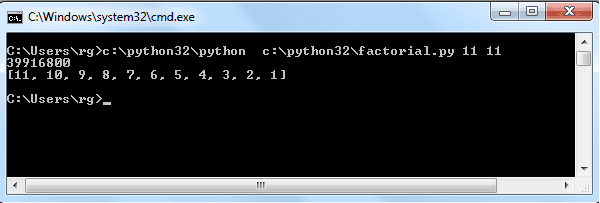Modules Path:

• In the directory ,the script is saved or in the current directory.
• in PYTHONPATH (a list of directory names), default search path for module files. PYTHONPATH is an environment variable. You will get it using 'env' command in UNIX-based operating system or in the properties of My Computer in windows system.
• the installation-dependent default.

Standard Module:

Python comes with numerous modules.

Python has many standard modules as a library. These are aimed to add efficiency or to access operating system primitives. Some of the modules depend upon the operating system.

sys is a Python Standard module which is very useful. It is built into every Python interpreter.

The dir() function:

The built-in function dir() is used to get the names (a sorted list of strings), a module is defined. Check it into Python shell.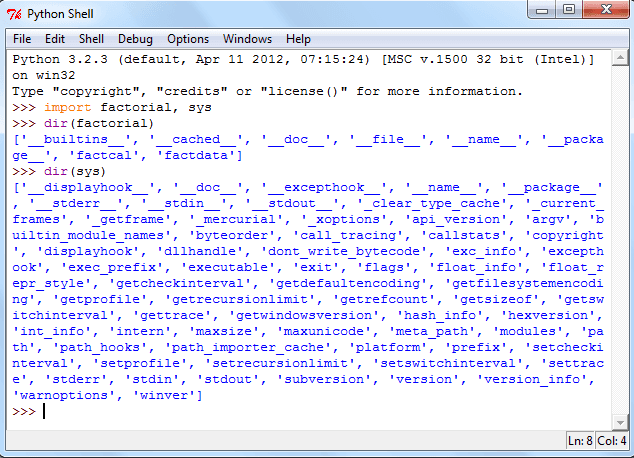Previous: Python User define function
Next: Home - Python Calendar Module

Test your Python skills with w3resource's quiz

﻿

## Python: Tips of the Day

Curries a function:

Example:

```from functools import partial

def tips_curry(fn, *args):
return partial(fn,*args)
add = lambda x, y: x + y
```100